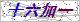# 当前位置：# 产品展示Products# 光面熔喷滤芯应用

 型    号： 所属分类： 光面熔喷滤芯 报    价： 市场价:分享到：

# 光面熔喷滤芯应用产品概述：

1.保安过滤；           2.药用试剂、用水等除杂过滤；

 滤材 聚丙烯(PP) 中心杆/端盖 聚丙烯 外径 63mm、115mm 内径 28mm、30mm

工作条件

 PH值 1-13 zui高工作温度 65℃ zui大工作压差 2.0bar/21℃

订货信息

 型号 外径 过滤精度 长度 端盖形式 密封材料 中心杆 DLPP blank=63mm 1=1um 975=9.75" Blank=两开(无端盖) S=硅橡胶 Blank=无中心杆 BB=115mm 3=3um 987=9.87" F=两开(加垫片) E=乙丙橡胶 C=有中心杆 5=5um 10=10" M=222/平尾 B=丁晴橡胶 10=10um 20=20" P=222/翅片 V=氟橡胶 25=25um 30=30" T=226/平尾 50=50um 40=40" Q=226/翅片 75=75um 50=50" 100=100um 60=60" 150=150um

### 留言框

• #### 验证码：请输入计算结果（填写阿拉伯数字），如：三加四=70571-63253538

15867199277